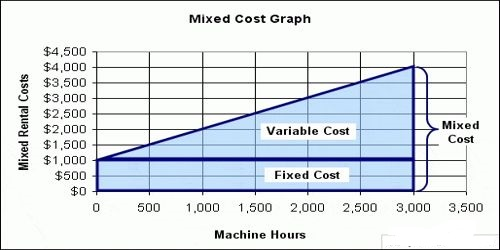# Elements of Mixed Cost

Elements of mixed costs: Mixed costs contain elements of both fixed and variable cost behavior. As with step costs, the fixed elements is determined by the planned range of activity level. Unlike step costs, though, usually, in a mixed cost, there is only one relevant range of activity and one level of fixed cost. It is important to understand the mix of these elements of a cost so that one can predict how costs will change with different levels of activity. The variable costs element of the mixed costs is a purely variable cost that varies proportionately with activity within the single relevant range. In a mixed cost, the variable cost is incurred in addition to fixed cost is the total mixed cost is the sum of the fixed cost plus the variable cost.

Total Mixed Costs = Total Fixed Cost \$ + (Variable Cost \$ per activity x # of the activity).

For example, if a company owns a production line, the total cost of that equipment in a month is a semi-variable cost. The fixed cost of the production line is \$10,000 per month, while the variable cost of utilities is \$150 per hour. If the production line runs for 160 hours per month, then the semi-variable cost calculation is: \$34,000 Total cost = \$10,000 fixed cost + (\$150/hour x 160 hours)